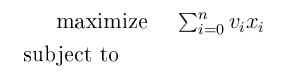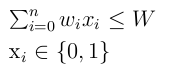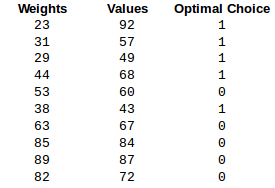## Tuesday, August 5, 2014

### Lecture on Recommender Systems

Great lecture on Recommender Systems by  Xavier Amatriain,  Researcher on Netflix.

# https://www.youtube.com/watch?v=bLhq63ygoU8

## Wednesday, July 16, 2014

### Genetic Algorithm for Knapsack using Hadoop

Development of Genetic Algorithm using Apache Hadoop framework to solve optimization problems

Introduction

This project I developed during a course on my Master intends to construct a Genetic algorithm to solve optimization problems, focusing on the Knapsack Problem. It uses as base the distributed framework Apache Hadoop.
The idea is to show that the MapReduce paradigm implemented by Hadoop is a good fit for several NP-Complete optimization problems. As knapsack, many problems present a simple structure and converge to optimal solutions given a proper amount of computation.

Genetic Algorithm

The algorithm was developed based on a Genetic paradigm. It starts with a initial random population (random instances to the problem). Then, the best individuals are selected among the population (instances that generate the best profits for the knapsack). A phase of crossover was then implemented to generate new instances as combination of the selected individuals. In this phase, parts of each selected individual are randomly selected to form a new instance.
The next step of the process is the mutation phase. In this phase, “genes” are selected also randomly, and changed. In the knapsack context this means that random items are substituted from the solutions found.
This cycle can be repeated several times, until good individuals are generated.
The steps are reproduced below:

Initial Population → Selection → Crossover → Mutation

The Knapsack ProblemThe goal is to maximize the objective function, where vi represents the value of each element i, and variable xi represents whether element i is going to be taken or not. The maximization has to take into account the weight of each element represented by the first constraint.

This problem was chosen because its solution only require enough amount of processing, and this processing can be done in parallel. The space of possible solutions can be seen as a set of local maximums that are going to be tested by the genetic algorithm. Hopefully, with enough computation, the global maximum can be achieved.

Validation Results

To demonstrate that the algorithm find a correct result with high probability, it was tested against two validation datasets. One was composed of a set of 10 items and another with 15 items.

With the validation input P01, we have:

P01 is a set of 10 weights and profits for a knapsack of capacity 165.The optimal result value is 309, by selecting items 0, 1, 2, 3 and 5 .

The algorithm finds the correct answer:

Best 5 answers:

Value Items Selected
270 0 1 2 9
276 0 2 3 6
277 0 1 3 4
284 0 1 3 6
309 0 1 2 3 5

The input P02 has optimal profit value 1458, and the algorithm also finds the correct answer.

These dataset were found on:

Setting up the Hadoop Cluster on a Single Machine

For testing purposes, here follows how to start on Hadoop, and set it up on a single machine.

Download hadoop 1.21 from:

http://hadoop.apache.org/releases.html

Install a Java 6:

\$ sudo apt-get install oracle-java6-installer

Inside the hadoop home directory, update a configuration file in conf/ :

\$ vim conf/hadoop-env.sh

export JAVA_HOME=/usr/lib/jvm/java-6-oracle/
export HADOOP_HOME=/home/renata/hadoop-1.2.1
export HADOOP_VERSION=1.2.1

Create an input directory, to place your input file:

\$ mkdir input

Put some content in:

\$ cp conf/*.xml input

To test if your hadoop is working properly:

\$ bin/hadoop jar hadoop-examples*.jar grep input output 'dfs[a-z.]+'

Don't forget to remove the output file after running a Job. Hadoop doesn't do this for you.

\$ rm -rf output

Now, to test the Genetic jar file:

\$ export HADOOP_HOME=/home/renata/hadoop-1.2.1
\$ export HADOOP_VERSION=1.2.1

To compile the code run :

\$ javac -classpath \${HADOOP_HOME}/hadoop-core*.jar -d genetic_classes Genetic.java

And create the jar:

\$ jar -cvf genetic.jar -C genetic_classes/ .

Now, to run the code once you put the input data on "input" directory:

\$ bin/hadoop jar genetic.jar org.myorg.Genetic input output

PS: A large large dataset consisting of 10.000 items, with optimal profit value of 7145 and a weight capacity of 431 can be found on: https://github.com/jaredtking/knapsack-lisp/blob/master/datasets/large01.

## Friday, January 31, 2014

### Dealing with NP-Hard Problems: An Introduction to Approximation Algorithms

This is just a quick overview on approximation algorithms. It is a broad topic to discuss. For more information go to References.

The famous NP-Complete class is known for its possible intractability. NP means non deterministic polynomial and for a problem to be NP-Complete it has to be
•   NP  (verified in polynomial time) and
•   NP-Hard (as hard as any other problem in the NP class).

Among the several important problems that are NP-Complete or NP-Hard (on its optimization form) we can name the Knapsack, the Travel Salesmen, and the Set Cover problem.

Even though no efficient optimal solution might exist for NP-Complete problems we still need to address this issue due to the amount of practical problems existent in the NP-Complete class.

Considering that even for medium volumes of data exponential brute-force is impractical, the option is abdicating the optimum solution as minimum as possible and pursuing an efficient algorithm.

Approximation algorithms are a way to deal with NP-Hard problems. They are polynomial-time algorithms, that return guaranteed near optimal solutions for any instance of the problem. The objective is to make the output as close to the optimal value as possible. We say that an α-approximation, is an algorithm that is within a α distance from the optimal value.

### Measures of Approximation

Among the measures of approximation it is possible to highlight:
• Absolute approximation
• Approximation factor
We say that an algorithm A is an absolute approximation for an instance I if it stays under a absolute distance k of the optimum algorithm OPT:

|A(I) − OPT(I)| ≤ k  ∀ instance I.

An algorithm has an approximation factor of α if:

A(I) ≤ α · OPT(I) ∀I, for minimization
A(I) ≥ α · OPT(I) ∀I, for maximization

being that α>1 for minimization and α<1 for maximization problems.

### Approximation Techniques

Greedy approaches are a highly intuitive and usually easy to implement approximation algorithm. It basically consists of making the "best" local choice at each step. For example, for the Set Cover problem, a greedy algorithm would be:

"At each round choose the set that minimizes the ratio weight of its weight to the number of currently uncovered elements it contains."

You can check a proof of approximation here .

Another way to create an approximation is by making a linear relaxation of a integral program. Remembering that many problems can be written in a more  mathematical form, were we have a objective function to maximize (or minimize), constraints and variables.
The Knapsack is a good example:

The goal here is to maximize the objective function, where vi represents the value of each element i, and variable xi represents whether element i is going to be taken or not. The maximization has to take into account the weight of each element represented by the first constraint.

Approximation through linear relaxation can be done in a very simple way. While Integral programming is NP-Hard, linear programming is not. If we relax (substitute) the constraint
to

we can have a solution (not optimal!) in polynomial time.

Here is what is would look like:

The linear relaxation serves as a lowerbound of the optimal solution in minimization problems and upperbound in maximization problems.

There is also a very powerful method called Primal-dual schema.

It is well known that, in some cases, optimal solutions satisfy the

The Primal-Dual schema works with a relaxed version of the complementary slackness conditions. It starts with a integral feasible solution for the primal and a feasible solution for the dual, for example. Iteratively, it satisfies the slackness conditions so that no constraint problem is broken in the process. When all conditions are satisfied the program ends.

It can be a little complex, so I am going to do a post about it on the future. For now, to understand it in details please read .

### Classes of Approximation

Algorithms as the mentioned above can be classified by their approximation "degree", or how much it is possible to approximate them of the optimal solution.
Following there are listed the classifications:

• PO - problems for which there are exact polynomial algorithms. This an extension of the P class to optimization problems.
• PTAS (Polynomial Time Approximation Scheme) -  It presents an approximation scheme which is a (1 + e)OPT approximation for minimization, and, (1 - e)OPT for maximization, where e is a relative error (rational number).
• FPTAS  (Fully Polynomial Time Approximation Scheme) -  as PTAS, FPTAS is also a polynomial scheme, but FPTAS running time is polynomial in the inverse of the error (1/e).
• APX -  there is at least one polynomial α-approximation (for some constant α).
• NPO - is an extension of the NP class to optimization problems. This means that an algorithm A is in the NP Optimization class if its instances are polynomial in size,  and solution for the problem can be verified in polynomial time and the size of the objective function (solution) can be calculated in polynomial time.
This is the relation among the classes:

PO ⊆  FPTAS ⊆ PTAS ⊆ APX ⊆ NPO

If an algorithm is in the FTPAS class, this usually means that it can have better approximations than one in the APX class.

The knapsack problem, for example, has been proved to be in FPTAS . If by any chance someone proved it to be also in any other class of the above, than P=NP.

### References

 D. P. Williamson and D. B. Shmoys. The Design of Approximation Algorithms. 2010. Cambridge University Press

 V. Vazirani. Approximation Algorithms. 2001. Springer-Verlag.

 (PORTUGUESE) M.H. Carvalho, M.R. Cerioli, R. Dahab, P. Feofiloff, C.G. Fernandes, C.E. Ferreira, K.S. Guimarães, F.K. Miyazawa, J.C. Pina Jr., J. Soares, Y. Wakabayashi. Uma introdução sucinta a algoritmos de aproximação. 23o Colóquio Brasileiro de Matemática, IMPA, Rio de Janeiro.

  Giorgio Ausiello, Pierluigi Crescenzi, and Marco Protasi. Approximate Solution of NP Optimization Problems. Theor. Comput. Sci. 150(1):1-55 (1995)